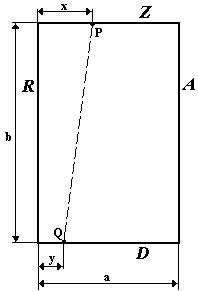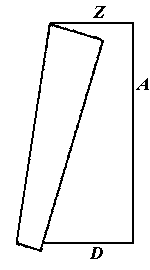시간 제한메모리 제한제출정답맞힌 사람정답 비율
1 초 128 MB22000.000%

## 문제

Edges of a rectangular sheet of paper are marked by letters A,R,Z,D. The length of the edges Z and D is a , while that of edges A and R - b centimeters.

On the side Z, x centimetrs from the side R, the point P is dotted, but on the side D, y centimeters from the side R, the point Q is dotted (see Figure 1)

The sheet is folded so that the folding line coincides with the segment PQ (see Figure 2).

You are to calculate the area covered by the folded sheet.Figure 1Figure 2

## 입력

Integers a (0 < a ≤ 1000), b (0 < b ≤ 1000), x (0 ≤ x ≤ a), y (0 ≤ y ≤ a) are inputed from the keyboard.

## 출력

On the screen the area covered by the folded sheet (in square centimeters) with at least 6 significant digits must be written.

## 예제 입력 1

7 100 1 1


## 예제 출력 1

600.000


## 예제 입력 2

13 5 10 3


## 예제 출력 2

51.7857